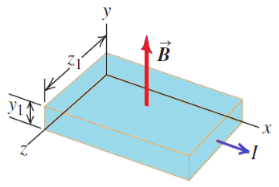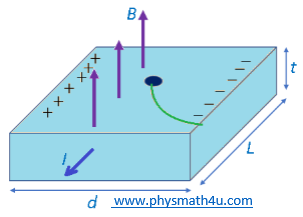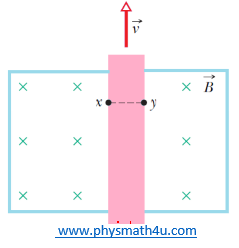# The Hall Effect (Crossed Fields) Problems and Solutions

Problem#1

Figure 1 shows a portion of a silver ribbon with z1 = 11.8 mm and y1 = 0.23 mm carrying a current of 120 A in the +x-direction. The ribbon lies in a uniform magnetic field, in the y-direction, with magnitude 0.95 T. If there are 5.85 x 1028 free electrons per cubic meter, find (a) the magnitude of the drift velocity of the electrons in the x-direction; (b) the magnitude and direction of the electric field in the z-direction due to the Hall effect; (c) the Hall emf.Fig.1

Known:
Thickness of silver ribbon, y1 = 0.23 mm = 0.23 x 10-3 m
wide of silver ribbon, z1 = 11.8 mm = 11.8 x 10-3 m
a current of I = 120 A
Uniform magnetic field, B = 0.95 T
Charge carriers per unit volume of copper = 5.85 x 1028 electrons/m3

(a) the magnitude of the drift velocity of the electrons in the x-direction is

vd = I/[y1z1nq]
vd = 3.0 A/[0.23 x 10-3 m x 11.8 x 10-3 m x 5.85 x 1028 electrons/mx 1.6 x 10-19 C]
vd = 4.7 x 10-3 m/s

(b) the magnitude and direction of the electric field in the z-direction due to the Hall effect is

vd = E/B
then,
E = Bvd = 0.95 T x 4.7 x 10-3 m/s = 4.5 x 10-3 N/C, in the +z-direction.

(c) the Hall emf is potential difference between the two edges of the strip (at z = 0 and z = z1) that result from the electric field calculated in part (b)

εhall = Ez1 = (4.5 x 10-3 N/C)(11.8 x 10-3 m) = 5.3 x 10-5 V

Problem#2
Let Fig. 1 represent a strip of an unknown metal of the same dimensions as those of the silver ribbon in Problem#1. When the magnetic field is 2.29 T and the current is 78.0 A, the Hall emf is found to be 131 μV. What does the simplified model of the Hall effected in this section give for the density of free electrons in the unknown metal?

Known:
a current of I = 78.0 A
Uniform magnetic field, B = 2.29 T
emf is V = 131 μV = 131 x 10-6 V
Area A = y1z1

the electric field, E = ε/z1 and │q│ = e.
The number density of charge carriers are measured by

n = ByIz1/Aqε = BzI/y1qε
n = [78.0 A x 2.29 T]/[2.3 x 10-4 m x 1.6 x 10-19 C x 1.31 x 10-6 V]
n = 3.7 x 1028 electrons/m3

Problem#3
In a Hall-effect experiment, a current of 3.0 A sent length wise through a conductor 1.0 cm wide, 4.0 cm long, and 10 mm thick produces a transverse (across the width) Hall potential difference of 10 μV when a magnetic field of 1.5 T is passed perpendicularly through the thickness of the conductor. From these data, find (a) the drift velocity of the charge carriers and (b) the number density of charge carriers. (c) Show on a diagram the polarity of the Hall potential difference with assumed current and magnetic field directions, assuming also that the charge carriers are electrons.

Known:
a current of I = 3.0 A
wide of conductor d = 1.0 cm = 1.0 x 10-2 m
length of conductor, L = 4.0 cm = 4.0 x 10-2 m
Thickness of conductor, t = 10 μm = 1.0 x 10-6 m
Uniform magnetic field, B = 1.5 T
Hall potential difference of V = 10 μV = 10 x 10-5 V

(a) the drift velocity of the charge carriers is

vd = E/B
with E is the electric field and can be written in term of the potential difference as;
E= V/d
Then,
vd = V/dB = (10 x 10-5 V)/[1.0 x 10-2 m x 1.5 T] = 6.7 x 10-4 m/s

(b) the number density of charge carriers is

n = Bi/Vte = I/(etdvd)
n = 3.0 A/[1.6 x 10-19 C x 10 x 10-6 m x 1.0 x 10-2 m x 6.7 x 10-4 m/s]
n = 2.8 x 1029/m3

(c) Diagram the polarity of the Hall potential difference with assumed current and magnetic field directions, assuming also that the charge carriers are electrons shown in the figure 2.Fig.2
Problem#4
A strip of copper 150 mm thick and 4.5 mm wide is placed in a uniform magnetic field B of magnitude 0.65 T, with B perpendicular to the strip. A current i = 23 A is then sent through the strip such that a Hall potential difference V appears across the width of the strip. Calculate V. (The number of charge carriers per unit volume for copper is 8.47 x 1028 electrons/m3.)

Known:
Thickness of copper strip, t = 150 μm = 150 x 10-6 m
Width of copper strip, w = 4.5 mm = 4.5 x 10-3 m
Uniform magnetic field, B = 0.65 T
Current in the strip, i = 23 A
Charge carriers per unit volume of copper = 8.47 x 1028 electrons/m3

The number density of charge carriers are measured by

n = Bi/Vte

Then, potential difference is

V = Bi/net
V = [0.65 T x 23 A]/[8.47 x 1028 electrons/m x 1.6 x 10-19 C x 150 x 10-6 m]
V = 7.35 x 10-6 V = 7.35 μV

Problem#5
A metal strip 6.50 cm long, 0.850 cm wide, and 0.760 mm thick moves with constant velocity v through a uniform magnetic field B = 1.20 mT directed perpendicular to the strip, as shown in Fig. 3. A potential difference of 3.90 μV is measured between points x and y across the strip. Calculate the speed v.Fig.3
Known:
Thickness of metal strip, t = 0.760 mm = 0.76 x 10-3 m
Width of metal strip, w = 0.850 cm = 0.85 x 10-2 m
Uniform magnetic field, B = 1.20 mT = 1.20 x 10-3 T
A potential difference of V = 3.90 μV = 3.90 x 10-6 V

For a free charge q inside the metal strip wirh velocity we have

qE B

We set this force equal to zero and use relation between (uniform) electric field and potential difference.
Then, the speed v is

v = E/B

with E is the electric field and can be written in term of the potential difference as;

E= │Vx – Vy│/dxy

Hence,
v = │Vx – Vy│/Bdxy
v = 3.90 x 10-6 V/[1.20 x 10-3 T x 0.850 x 10-2 m]
v = 0.382 m/s